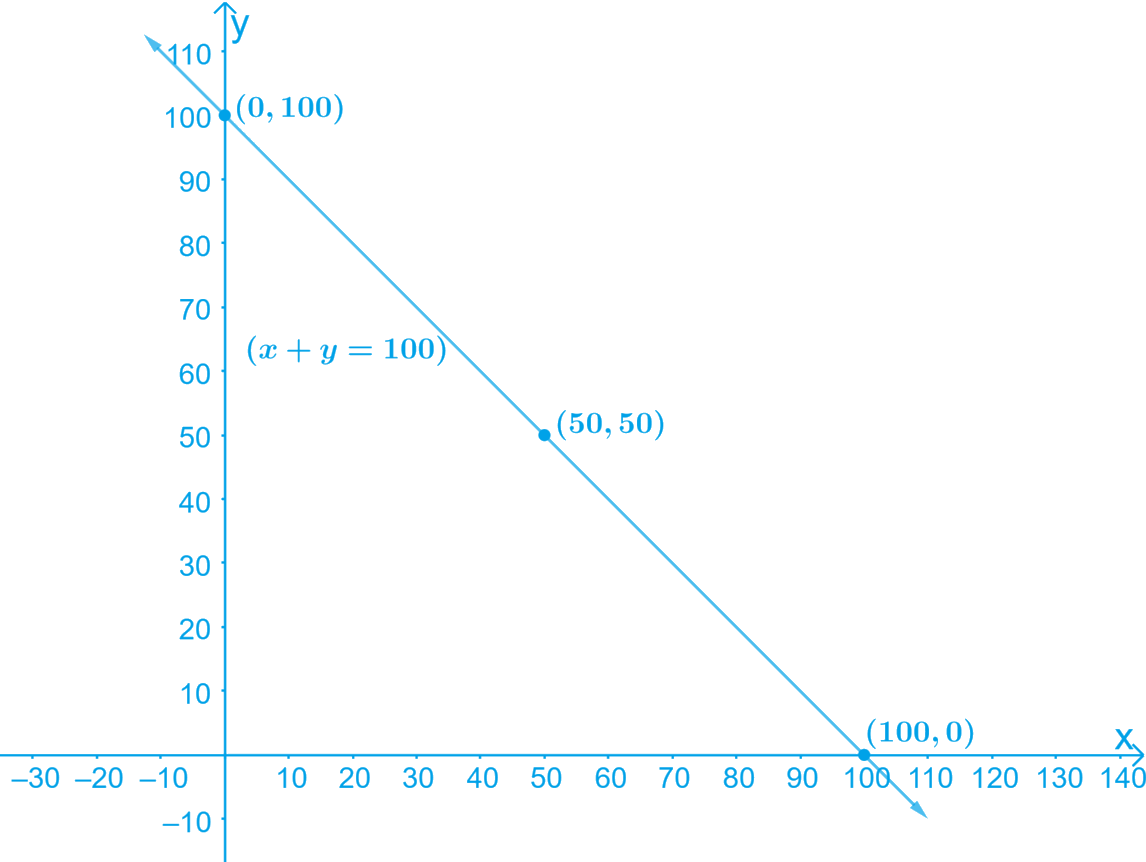# Ex.4.3 Q7 Linear Equations in Two Variables Solution - NCERT Maths Class 9

Go back to  'Ex.4.3'

## Question

Yamini and Fatima, two students of Class $$IX$$ of a school, together contributed $$₹\, 100$$ towards the Prime Minister’s Relief Fund to help the earthquake victims. Write a linear equation which satisfies this data. (You may take their contributions as ₹ $$x$$ and ₹ $$y$$.) Draw the graph of the same.

Video Solution
Linear Equations In Two Variables
Ex 4.3 | Question 7

## Text Solution

What is known?

Yamini and Fatima together contributed $$₹\, 100$$

What is Unknown?

Yamini and Fatima contributions and graph for the same.

Reasoning:

We can let Yamini and Fatima contributions as $$₹$$ $$x$$ and $$₹$$ $$y$$ and form linear equation. Then find $$x$$ and $$y$$ value to draw graph.

Steps:

• Let the amount that Yamini and Fatima have contributed individually be $$₹$$ $$x$$ and $$₹$$ $$y$$ respectively towards the Prime Minister’s Relief fund.
• The total amount contributed by Yamini and Fatima together is $$\rm = ₹\, 100$$

Therefore, $$x + y = 100$$

On transposing, we get $$y = 100 \,– x$$

This is a linear equation in two variables of the form $$ax + by + c = 0$$

Let us consider this as,

$$y = 100\, – x \quad \dots \text{ Equation } (1)$$

By substituting different values of $$x$$ in the Equation (1), we get different values for $$y$$

• When $$x = 0, y = 100$$
• When $$x = 50, y = 50$$
• When $$x = 100, y = 0$$

Thus, we have the following table with all the obtained values of $$x$$ and $$y$$:

 $$x$$ $$0$$ $$50$$ $$100$$ $$y$$ $$100$$ $$50$$ $$0$$

By Plotting the points $$(0, 100), (100, 0)$$ and $$(50, 50)$$ on the graph paper and drawing a line joining them, we obtain the required graph.

The graph of the line represented by the given equation is as shown.Here, variable $$x$$ and $$y$$ are representing the amount contributed by Yamini and Fatima respectively and these quantities cannot be negative. Hence, only those values of $$x$$ and $$y$$ which are lying in the $$1\,\rm st$$ quadrant are considered.

Learn from the best math teachers and top your exams

• Live one on one classroom and doubt clearing
• Practice worksheets in and after class for conceptual clarity
• Personalized curriculum to keep up with school